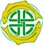﻿ Principal Component Analysis (PCA)Principal Component Analysis (PCA)
 Principal Component Analysis (PCA) is the oldest and still one of the most frequently-used ordination techniques in community ecology. It is most appropriate for full quantitative data, but can be used if abundance is classified into a number of abundance classes. The objective of the method is to express the relationship between the samples in a 2- or 3-dimensional space that can be plotted and usefully visualised. This can only be achieved if many of the variables are positively or negatively correlated. Normally this will be so for a number of reasons. First, there is the interdependence between organisms in an ecosystem, and second, because many variables respond similarly to environmental variables such as temperature and water.   General descriptions of the procedure for biologists are given Legendre & Legendre (1998); Digby & Kempton (1987); Kent & Coker (1992).   The analysis is undertaken on either the between-sample variance-covariance matrix, or the correlation matrix. If the variables vary greatly in abundance you will probably need to transform the data by taking logarithms or using a square-root transformation. Logarithmic transformations would be excellent if it were not for the fact that zeros cannot be handled. A frequently-used procedure is to add 1 to all the observations. This can distort the output, and so it is probably more appropriate to use a square-root transformation.   If you undertake a PCA on the correlation matrix you will be giving all variables, irrespective of abundance, equal weighting, whereas the analysis undertaken on the variance-covariance matrix will reflect differences in abundance, but can result in the numerically-dominant variables determining the output. When successful, PCA will present major features of a complex community in only 2 or 3 dimensions and the ordination of samples (sites) along these new axes can be related to underlying environmental factors that are moulding community structure. PCA can be judged a success when the first two or three principal axes explain an appreciable proportion of the total variability in the data set. For large ecological data sets with > 20 species, if the three largest axes can explain more than 30% of the variance, this would generally be considered satisfactory.   If you wish to use R see Run R code